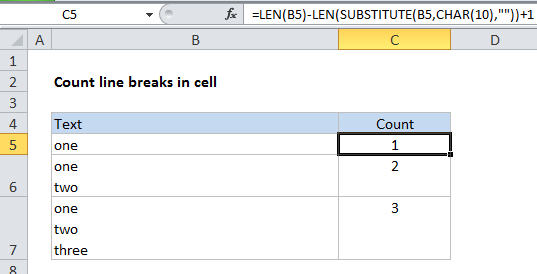## Excel Office

Excel How Tos, Tutorials, Tips & Tricks, Shortcuts

# How to count line breaks in cell in Excel worksheet

This tutorial shows how to count line breaks in cell in Excel worksheet using example below.

To count total lines in a cell, you can use a formula based on the LEN, SUBSTITUTE, and CHAR functions.

## Formula

`=LEN(B5)-LEN(SUBSTITUTE(B5,CHAR(10),""))+1`## Explanation

In the example, we are using this formula:

`=LEN(B5)-LEN(SUBSTITUTE(B5,CHAR(10),""))+1`

### How the formula works

First, the LEN function counts total characters in the cell B5.

Worked Example:   Nested IF function example in Excel

Next SUBSTITUTE removes all “line returns” from the text in B5 by looking for CHAR(10) which is the character code for the return character in Windows.  LEN returns the result inside of a second LEN, which counts characters without carriage returns.

Worked Example:   If cell is not blank in Excel

The second count is subtracted from the first, and 1 is added to the final result, since the number of lines is the number of returns + 1.

#### Mac version

On a Mac, the code for return character is 13 instead of 10, so use this formula instead:

`=LEN(B5)-LEN(SUBSTITUTE(B5,CHAR(13),""))+1`

#### Dealing with empty cells

The formula in the example shown will return 1 even if a cell is empty. If you need to guard against this problem, you can wrap the formula in IF statement like so:

`=IF(ISBLANK(B5),0,LEN(B5)-LEN(SUBSTITUTE(B5,CHAR(10),""))+1)`
Worked Example:   Roll back weekday to Friday base on a particular date in Excel